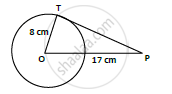# If Pt is a Tangent at T to a Circle Whose Center is O and Op = 17 Cm, Ot = 8 Cm. Find the Length of Tangent Segment Pt. - Mathematics

If PT is a tangent at T to a circle whose center is O and OP = 17 cm, OT = 8 cm. Find the length of tangent segment PT.

#### Solution

OP = 17cm

PT = length of tangent = ?T is point of contact. We know that at point of contact tangent and radius are perpendicular.

∴ OTP is right angled triangle ∠OTP = 90°, from Pythagoras theorem 𝑂𝑇2 + 𝑃𝑇2 = 𝑂𝑃2

82 + 𝑃𝑇2 = 172

PT sqrt(17^2 − 8^2) = sqrt(289 − 64)

=sqrt(225) = 15𝑐𝑚

∴ PT = length of tangent = 15 cm.

Concept: Concept of Circle - Centre, Radius, Diameter, Arc, Sector, Chord, Segment, Semicircle, Circumference, Interior and Exterior, Concentric Circles
Is there an error in this question or solution?

#### APPEARS IN

RD Sharma Class 10 Maths
Chapter 8 Circles
Exercise 8.2 | Q 1 | Page 33